## 随机数排序，最大值，最后写出 热

@曹思源 你好，想跟你请教个问题：

（1）对产生的N个随机数进行升序的排序；
（2）在N个随机数中找出最大值；
（3）在排序的随机数中插入一个数后，仍有序；
（4）将排序前后的数组写入到文件Sort_array.txt中。

0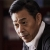```#include <iostream>
#include <fstream>
#include <iomanip>
using namespace std;
void print(int i_Array[],int count);                   //声明输出随机数的函数
int chackArray(int i_Value,int i_Array[],int count);   //声明检查产生的随机数是否存在函数
void getArray(int i_Array[],int count);                //声明获得随机数数组函数
void up_Sort(int i_Array[],int count);                 //声明升序排序函数
void down_Sort(int i_Array[],int count);               //声明降序排序函数
int chackArraySort(int i_Array[],int count);           //声明检查排序，如果返回值为0，则说明函数数组为无序排列，如果为1，则为升序排列，如果返回2，则为降序，如果返回3，则数组数据完全相同
void insert(int Array[] , int Number ) ;               //插入一个数字，插入后仍有序
void printChackSort(int Number);                       //根据返回值输出数组的性质
void writeIn( int Array[] ) ;                          //写入文件函数
int main()
{
const int count = 100;          //定义要输出的随机数的个数
int i_Array[count] = {0};		//初始化输出随机数的数组
print(i_Array,count);
printChackSort(chackArraySort(i_Array,count));
cout<<endl;

getArray(i_Array,count);		//获得随机数数组
print(i_Array,count);           //输出生成的随机数
printChackSort(chackArraySort(i_Array,count));
cout<<endl;

up_Sort(i_Array,count);         //升序排序
print(i_Array,count);           //输出升序排序后的随机数组
printChackSort(chackArraySort(i_Array,count));
cout<<endl;

down_Sort(i_Array,count);       //降序排序
print(i_Array,count);           //输出降序排序后的随机数组
printChackSort(chackArraySort(i_Array,count));
cout<<endl;

system("pause");                //暂停定屏
return 0;
}

//定义输出数组的函数
void print(int i_Array[],int count)
{
for(int i = 0; i < count;i++)
{
cout<<setw(5)<<i_Array[i];
if(i%10 == 9)
cout<<endl;
}
return;
}

//定义获得数组的函数，其中Number为数组中的第几个数字
void getArray(int i_Array[],int count)
{
int Number = 0;
for(int i =0;;i++)
{
int i_Value = rand()%100;                      //获得一随机数
if(chackArray(i_Value,i_Array,Number))     //检查是否在数组里存在，如果不存在则执行下面的过程否则跳过下面的过程
{
i_Array[Number] = i_Value;             //将获得的数字赋给数组中的第Number个数字
Number++;
if(Number == count )                   //如果获的数字个数达到要求，则返回，反之集训
return;
}

}
return;
}

//定义检查重合的函数，其中i_Value为要检查的数，i_Array[]为检查的数组范围,count为数组中的数的个数，如果被检查数不存在则返回1，否则返回0
int chackArray(int i_Value,int i_Array[],int count)
{
int result = 1;

for(int i = 0; i<count;i++)
{
if(i_Value == i_Array[i])
return 0;
}
return 1;
}

//定义升序排序函数，这里使用的是插入排序的排序方法
void up_Sort(int i_Array[],int count)
{
int i_Temp = 0;
for(int i = 1;i < count;i++ )
{
int j = i;
i_Temp = i_Array[i];
while(j > 0 && i_Temp < i_Array[j - 1])
{
i_Array[j] = i_Array[j - 1];
j--;
}
i_Array[j] = i_Temp;
}
return;
}

//定义降序排序函数，这里采用的是插入排序法
void down_Sort(int i_Array[],int count)
{
int i_Temp = 0;
for(int i = 1;i < count;i++ )
{
int j = i;
i_Temp = i_Array[i];
while(j > 0 && i_Temp >i_Array[j - 1])
{
i_Array[j] = i_Array[j - 1];
j--;
}
i_Array[j] = i_Temp;
}
return;
}

//检查数组的排序。
int chackArraySort(int i_Array[],int count)
{
int temp = 0;
int chackResult = 0;
if(sizeof(i_Array) == 1)
return 4;                                    //如果数组中只有一个元素，则返回4

if(i_Array == i_Array)                     //初次检查数组，前两个数的排序
temp = chackResult = 3;
else if(i_Array > i_Array)
temp = chackResult = 2;
else if(i_Array < i_Array)
temp  = chackResult = 1;

for(int i = 1;i < count -1;i ++)                 //根据之后的数字确定排序
{
if(i_Array[i] == i_Array[i + 1])
chackResult = 3;
else if(i_Array[i] > i_Array[i + 1])
chackResult = 2;
else if(i_Array[i] < i_Array[i + 1])
chackResult = 1;

if(chackResult != temp)
{
chackResult = 0;
return chackResult;
}
}
return chackResult;
}

//输出数组排序的方式
void printChackSort(int Number)
{
switch(Number)
{
case 1:
cout<<endl<<"    该数组为升序排序"<<endl;
break;
case 2:
cout<<endl<<"    该数组为降序排序"<<endl;
break;
case 3:
cout<<endl<<"    该数组的数据完全相同"<<endl;
break;
case 0:
cout<<endl<<"    该数组是无序排列"<<endl;
break;
default:
cout<<endl<<"    该数组有问题"<<endl;
break;
}
}

//排序插入。升序
void insert(int Array[] , int Number )
{
int num = 0 ;
int len = sizeof( Array )/sizeof(Array[ 0 ] ) ;
while( num <= len || Array[ num ] < Number )
{
num ++ ;
}
for( int i = len ; i > num ; i -- )
{
Array[ i + 1 ] = Array[ i ] ;
}
Array[ num ] = number ;
}
//写入文件
void writeIn( int Array[] )
{
int len =  sizeof( Array ) / sizeof( Array ) ;
ifstream infile( "Sort_array.txt",ios::out ) ;
if( ! infile )
{
cerr<< " 文件打开失败"<< endl ;
exit( 1 ) ;
}
for( int i = 0 ; i < len ; i ++ )
infile( Array[ i ] ) ;
infile.close() ;
}```

0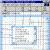0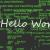```	function k_sort(\$arr){
\$num = count(\$arr);
if(\$num<=1){
return \$arr;
}
\$key = \$arr;
\$left = array();
\$right = array();
for(\$i=1; \$i<\$num; \$i++){
if(\$arr[\$i] <= \$key){
\$left[] = \$arr[\$i];
}else{
\$right[] = \$arr[\$i];
}
}
\$n++;
\$left = k_sort(\$left);
\$right = k_sort(\$right);
return array_merge(\$left,array(\$key),\$right);
}```

0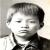#### 引用来自“HelloChina”的答案

```	function k_sort(\$arr){
\$num = count(\$arr);
if(\$num<=1){
return \$arr;
}
\$key = \$arr;
\$left = array();
\$right = array();
for(\$i=1; \$i<\$num; \$i++){
if(\$arr[\$i] <= \$key){
\$left[] = \$arr[\$i];
}else{
\$right[] = \$arr[\$i];
}
}
\$n++;
\$left = k_sort(\$left);
\$right = k_sort(\$right);
return array_merge(\$left,array(\$key),\$right);
}```

0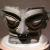#### 引用来自“HelloChina”的答案

```	function k_sort(\$arr){
\$num = count(\$arr);
if(\$num<=1){
return \$arr;
}
\$key = \$arr;
\$left = array();
\$right = array();
for(\$i=1; \$i<\$num; \$i++){
if(\$arr[\$i] <= \$key){
\$left[] = \$arr[\$i];
}else{
\$right[] = \$arr[\$i];
}
}
\$n++;
\$left = k_sort(\$left);
\$right = k_sort(\$right);
return array_merge(\$left,array(\$key),\$right);
}```

0#### 引用来自“HelloChina”的答案

```	function k_sort(\$arr){
\$num = count(\$arr);
if(\$num<=1){
return \$arr;
}
\$key = \$arr;
\$left = array();
\$right = array();
for(\$i=1; \$i<\$num; \$i++){
if(\$arr[\$i] <= \$key){
\$left[] = \$arr[\$i];
}else{
\$right[] = \$arr[\$i];
}
}
\$n++;
\$left = k_sort(\$left);
\$right = k_sort(\$right);
return array_merge(\$left,array(\$key),\$right);
}```

0#### 引用来自“HelloChina”的答案

```	function k_sort(\$arr){
\$num = count(\$arr);
if(\$num<=1){
return \$arr;
}
\$key = \$arr;
\$left = array();
\$right = array();
for(\$i=1; \$i<\$num; \$i++){
if(\$arr[\$i] <= \$key){
\$left[] = \$arr[\$i];
}else{
\$right[] = \$arr[\$i];
}
}
\$n++;
\$left = k_sort(\$left);
\$right = k_sort(\$right);
return array_merge(\$left,array(\$key),\$right);
}```

```    for (i = 0 ; i < n - 1; i++) {
for (j = i + 1; j < n ; j++){
if (a[i] > a[j]) {
int t = a[i] ; a[i] = a[j] ; a[j] = t;
}

}
}
}
//以上超传统

void sort(int *a,int n){
int i,j;
i = 0 ;
while (i < n - 1){
j = i+1;
while (j < n ){
if (a[i] > a[j]) {
int t = a[i] ; a[i] = a[j] ; a[j] = t;
}
j++;
}
i++;
}
}
//以上近似传统。
#define __GT_NODE(a,i,j) (a[i] > a[j])
#define __SWAP_NODE(a,i,j) do {int t = a[i]; a[i] =a[j];a[j] =t;}while (0)
void sort(int *a,int n){
int i,j;
i = 0 ;
while (i < n - 1){
j = i+1;
while (j < n ){
if (_GT_NODE(a,i,j)){
__SWAP(a,i,j);
}
j++;
}
i++;
}
}
//以上已经有模版的小样子了。再改
#define __GET_P(a,_T_) ((_T_*)(a))
#define __GT_NODE(a,i,j) (__GET_P(a,int)[i] > __GET_P(a,int)[j])
#define __SWAP_T(pa,pb,_T_) do {_T_ t = __GET_P(pa,_T_);\
__GET_P(pa,_T_) = __GET_P(pb,_T_); __GET_P(pb,_T_) = t;}while(0)
#define __SWAP_NODE_INT(a,i,j) __SWAP_T(a+i,a+j,int)
#define __GET_FIRST_BIAS(i) do {i = 0 ; } while (0)
#define __GET_NEXT(i) (i)+1
#define __NEXT_BIAS(i) do{i = __GET_NEXT(i);}while (0)
#define __CHECK_END(i,n)  ((i) < n)
void sort(void  *a,int n){//注意，此处已经不是int * 了，是void *，这样接口都可以统一。
int i,j;
__GET_FIRST_BIAS(i);
while (__CHECK_END(i,n-1)){
j = __GET_NEXT(i);
while (__CHECK_END(j,n) ){
if (_GT_NODE(a,i,j)){
__SWAP(a,i,j);
}
__NEXT_BIAS(j);
}
__NEXT_BIAS(i);
}
}
//以上已经有了类型拓展的方式。其实，对于任意数据结构，你可以通过修改#define

```#undef XXX
#define XXX
#include "templete.txtC"```00@一千年前的人 写代码真的很容易。。难的是数据结构的设计和规划。一不小心就整个算法要全部调整。毕竟算法除了自身逻辑外占比较少，额外的对数据结构的操作占很多。而且数据结构不一样，该结构的维护和相同算法处理方法也可能会有差异。